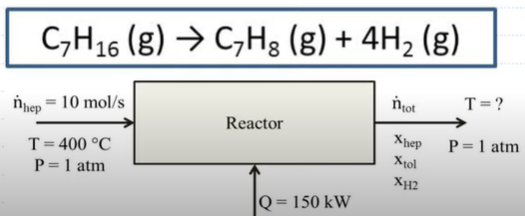#### Energy Balances with Reaction: Example Problems

Try to solve these problems before watching the solutions in the screencasts.

##### Example Problem 1

100 mol/h of N2 and 300 mol/h of H2 are fed to an isothermal reactor at 350°C to carry out the following gas-phase reaction:

N2 + 3H2 → 2NH3

Assume the heat capacities for the components are the following:

Cp(N2) = Cp(H2) = 29 J/mol-K

Cp(NH3) = 36 J/mol-K

How much heat must be removed from the reactor if the conversion is 75%? The heat of formation of ammonia at 25°C is -46 kJ/mol.

##### Example Problem 2

Consider the reaction of n-heptane to form toluene and hydrogen in a vapor-phase reactor. The fractional conversion of n-heptane is 0.7. The reaction requires 150 kW of heat to proceed. What is the outlet temperature? Assume negligible work and kinetic and potential energies.